# Volumetric Analysis Types of Titrimetric Methods Complexometric titrations

• Slides: 53Volumetric Analysis: Types of Titrimetric Methods Complexometric titrations A standard solution: is a reagent of exactly known concentration that is used in a titrimetric analysis Titration: is a process in which a standard reagent is added to a solution of an analyte until the reaction between the analyte and reagent is judged to be complete.Back-titration : is a process in which the excess of a standard solution used to consume an analyte is determined by titration with a second standard solution. Back-titrations are often required when the rate of reaction between the analyte and reagent is slow or when the standard solution lacks stability.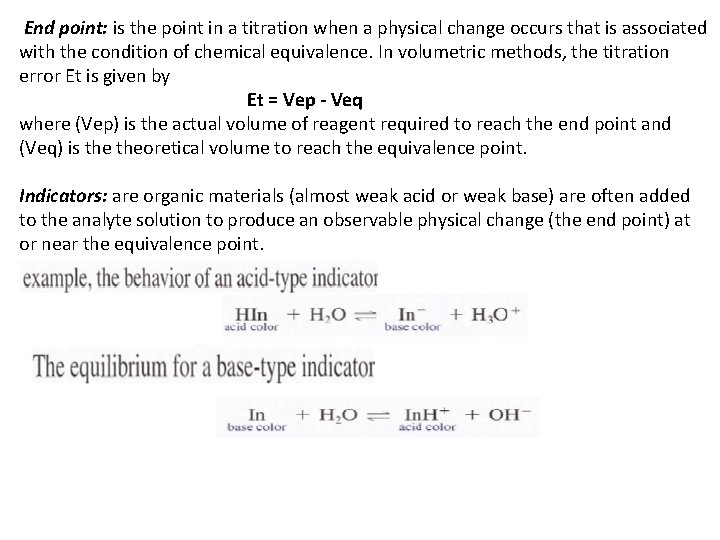End point: is the point in a titration when a physical change occurs that is associated with the condition of chemical equivalence. In volumetric methods, the titration error Et is given by Et = Vep - Veq where (Vep) is the actual volume of reagent required to reach the end point and (Veq) is theoretical volume to reach the equivalence point. Indicators: are organic materials (almost weak acid or weak base) are often added to the analyte solution to produce an observable physical change (the end point) at or near the equivalence point.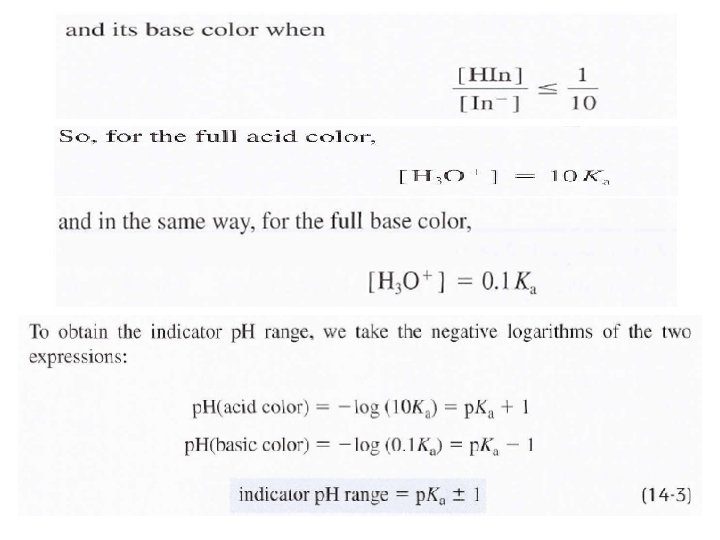Ph. ph Indicator for acid – base titration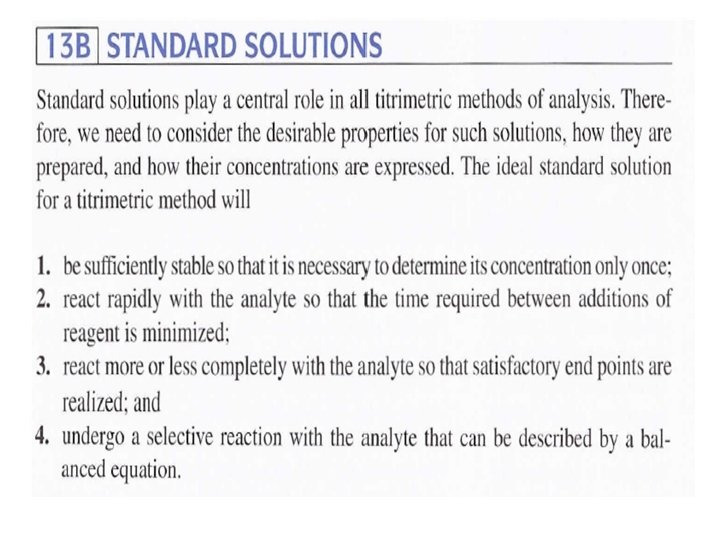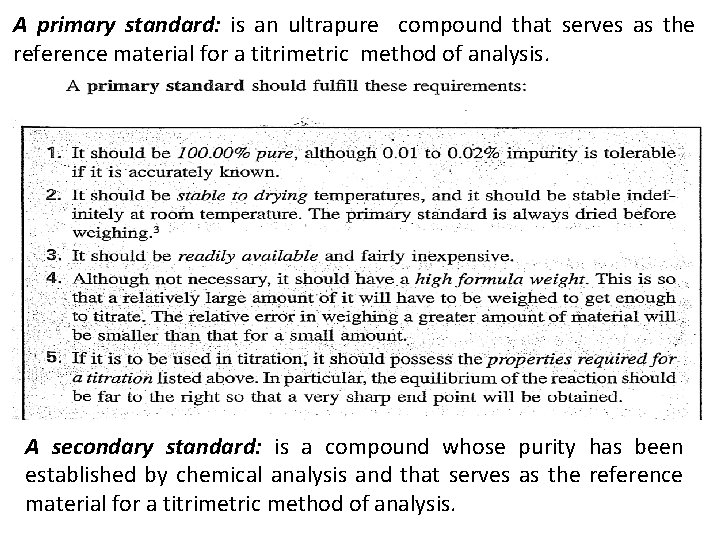A primary standard: is an ultrapure compound that serves as the reference material for a titrimetric method of analysis. A secondary standard: is a compound whose purity has been established by chemical analysis and that serves as the reference material for a titrimetric method of analysis.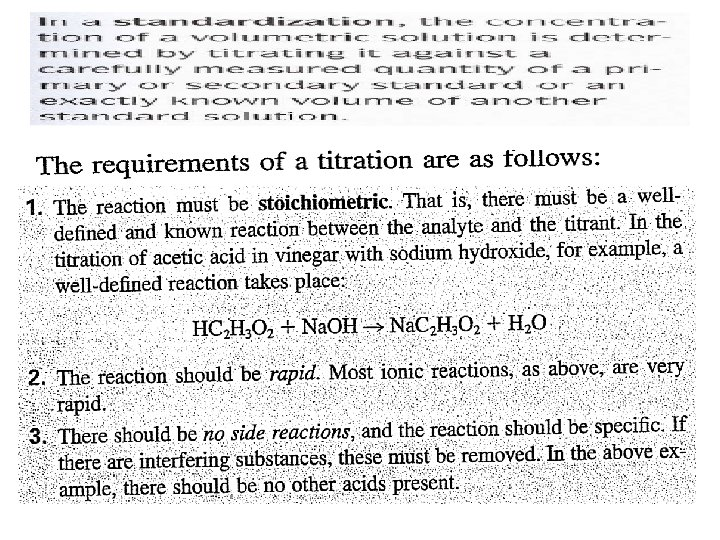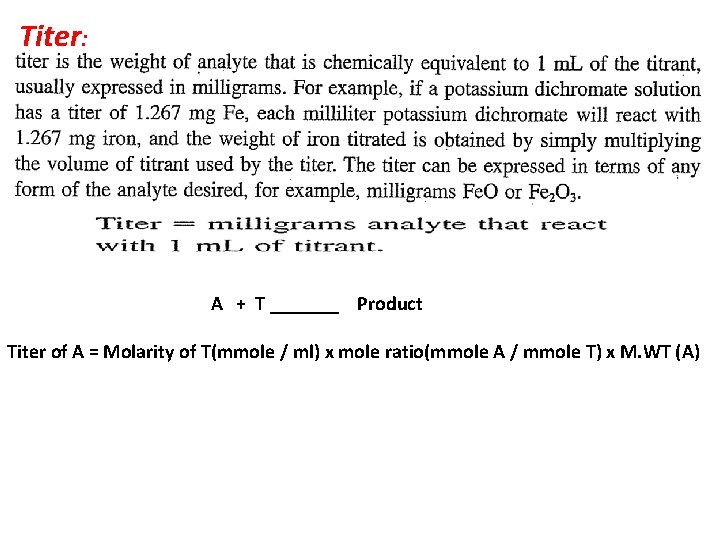Titer: A + T _______ Product Titer of A = Molarity of T(mmole / ml) x mole ratio(mmole A / mmole T) x M. WT (A)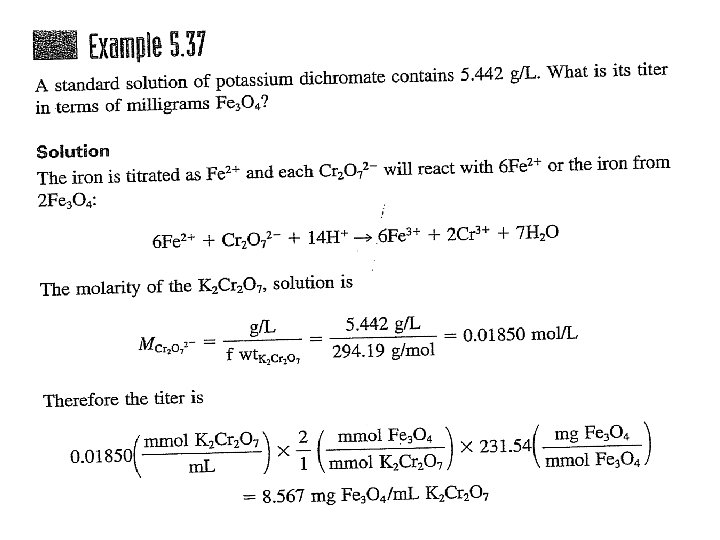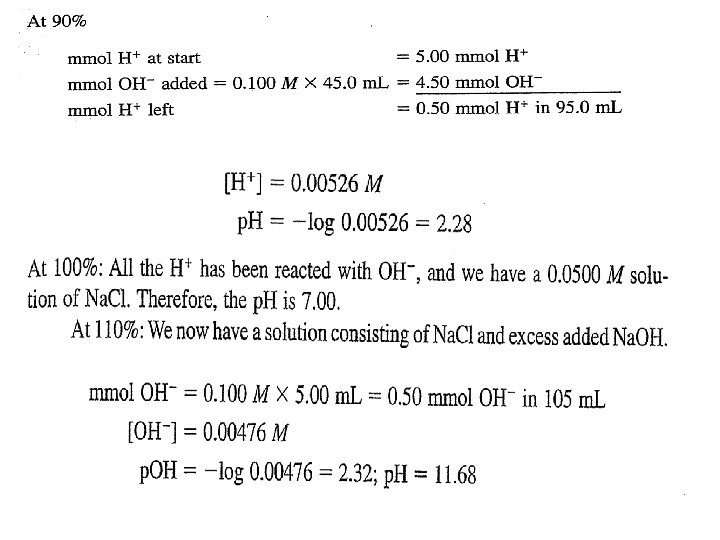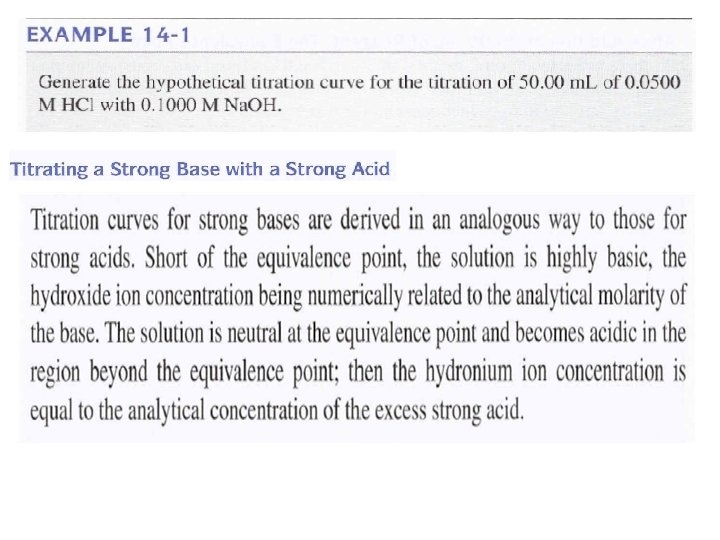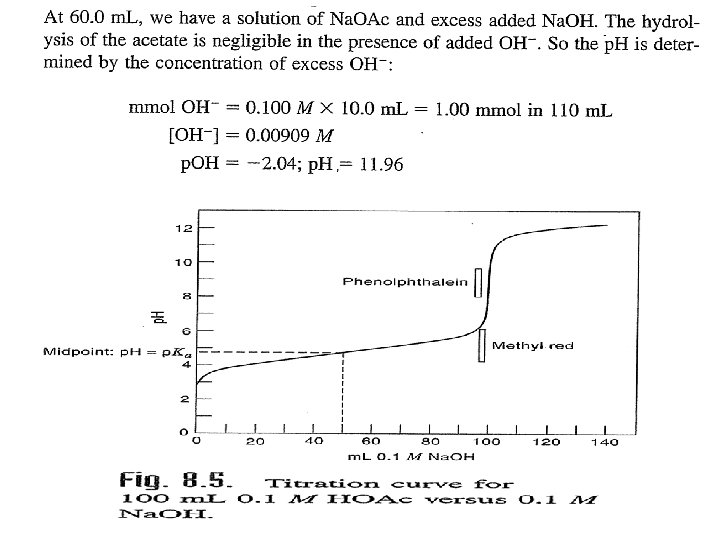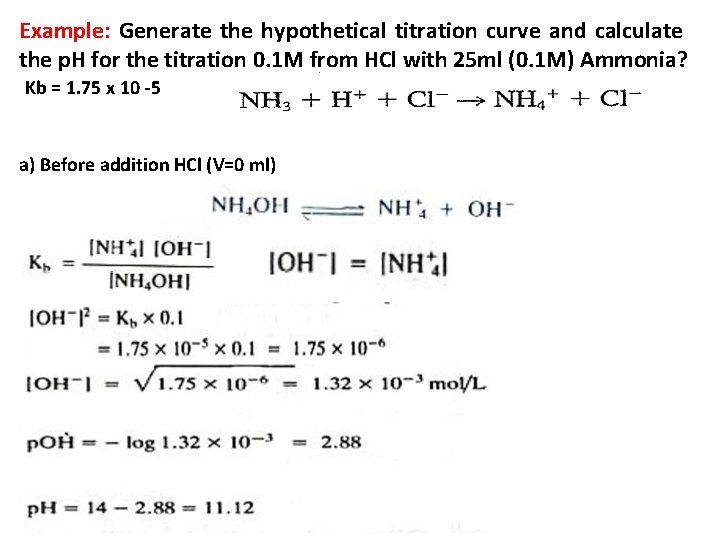Example: Generate the hypothetical titration curve and calculate the p. H for the titration 0. 1 M from HCl with 25 ml (0. 1 M) Ammonia? Kb = 1. 75 x 10 -5 a) Before addition HCl (V=0 ml)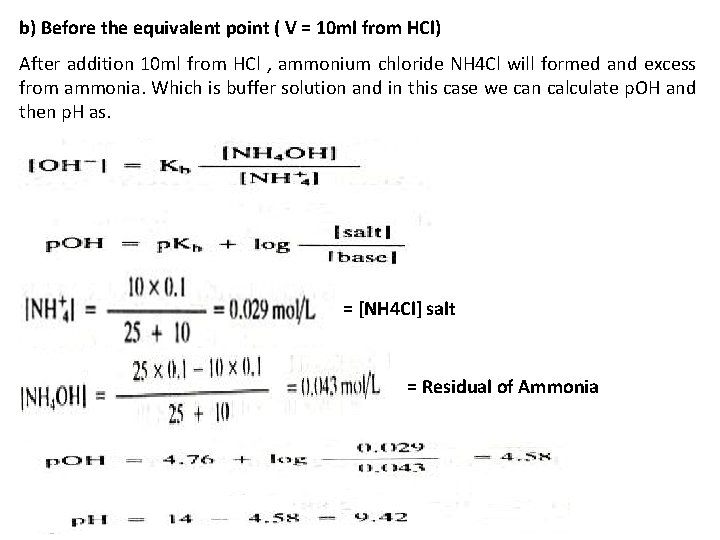b) Before the equivalent point ( V = 10 ml from HCl) After addition 10 ml from HCl , ammonium chloride NH 4 Cl will formed and excess from ammonia. Which is buffer solution and in this case we can calculate p. OH and then p. H as. = [NH 4 Cl] salt = Residual of Ammoniac) At the equivalent point: Which is mean addition equal volume (25 m) from HCl to 25 ml(0. 1 M) Ammonia to form salt of weak base only NH 4 Cl and we can calculate the p. H to the salt of weak base as. d) Post equivalent point: This case mean addition from HCl, like addition 26 ml. We can calculate the p. H from the excess of HCl as.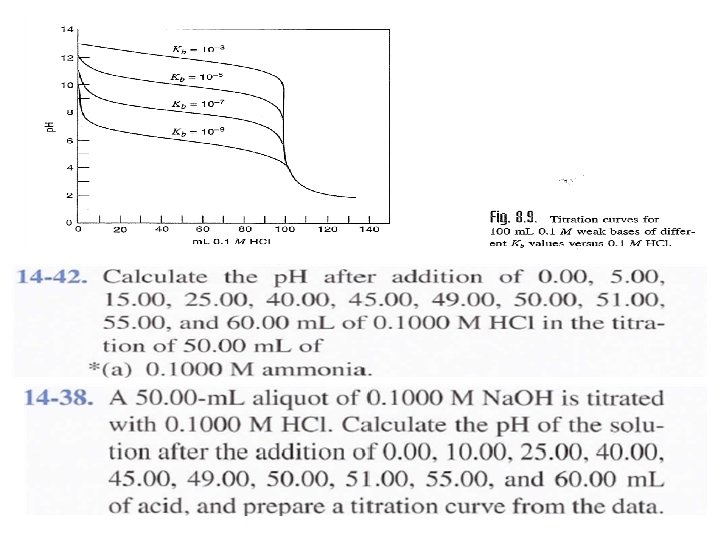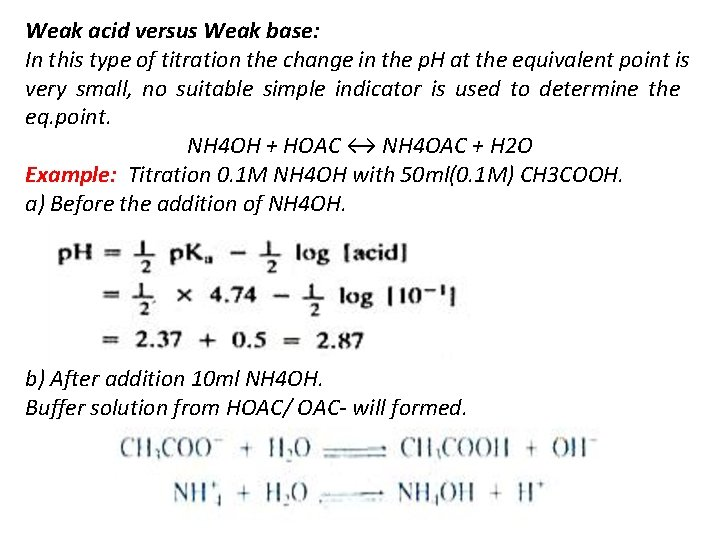Weak acid versus Weak base: In this type of titration the change in the p. H at the equivalent point is very small, no suitable simple indicator is used to determine the eq. point. NH 4 OH + HOAC ↔ NH 4 OAC + H 2 O Example: Titration 0. 1 M NH 4 OH with 50 ml(0. 1 M) CH 3 COOH. a) Before the addition of NH 4 OH. b) After addition 10 ml NH 4 OH. Buffer solution from HOAC/ OAC- will formed.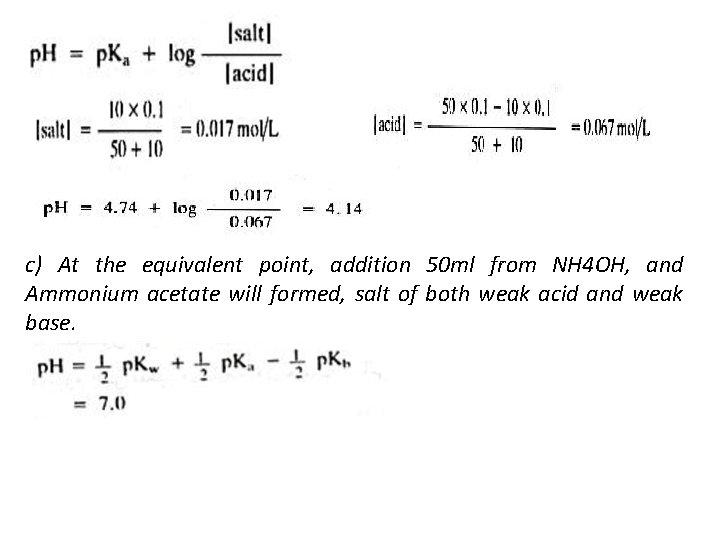c) At the equivalent point, addition 50 ml from NH 4 OH, and Ammonium acetate will formed, salt of both weak acid and weak base.d) Post equivalent point , after addition 60 ml from ammonia. That is mean another buffer solution will form that produce all HOAC were convert to Ammonium acetate , and the excess of ammonia.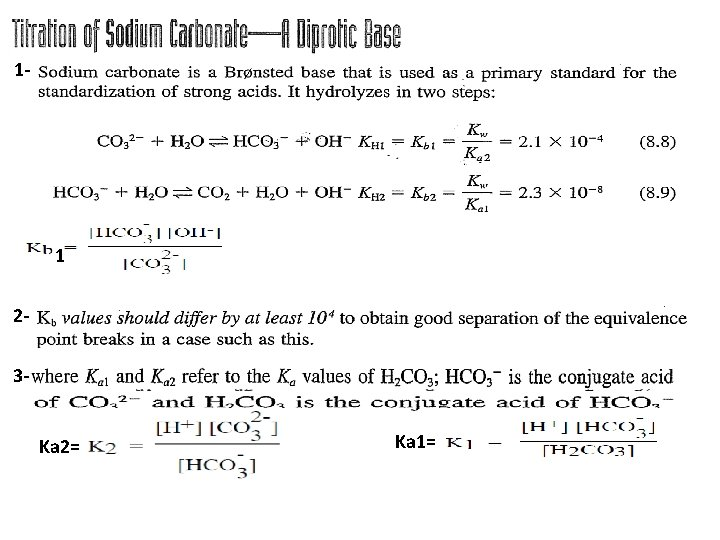1 - 1 23 Ka 2= Ka 1=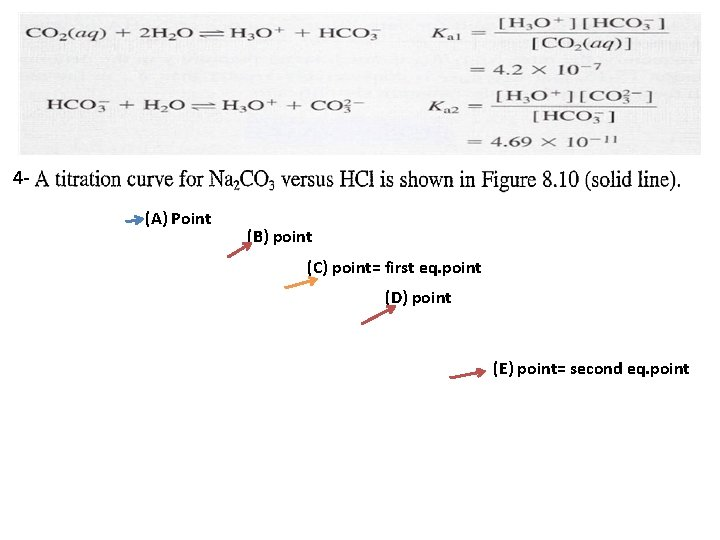4(A) Point (B) point (C) point= first eq. point (D) point (E) point= second eq. point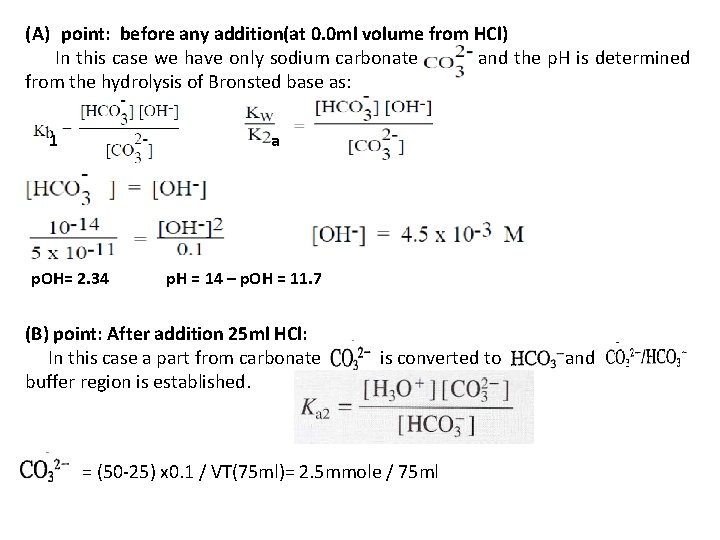(A) point: before any addition(at 0. 0 ml volume from HCl) In this case we have only sodium carbonate and the p. H is determined from the hydrolysis of Bronsted base as: 1 a p. OH= 2. 34 p. H = 14 – p. OH = 11. 7 (B) point: After addition 25 ml HCl: In this case a part from carbonate buffer region is established. is converted to = (50 -25) x 0. 1 / VT(75 ml)= 2. 5 mmole / 75 ml and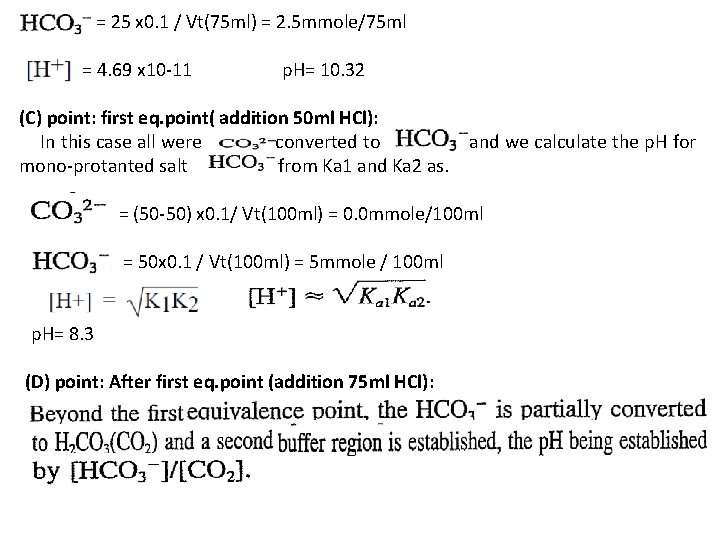= 25 x 0. 1 / Vt(75 ml) = 2. 5 mmole/75 ml = 4. 69 x 10 -11 p. H= 10. 32 (C) point: first eq. point( addition 50 ml HCl): In this case all were converted to and we calculate the p. H for mono-protanted salt from Ka 1 and Ka 2 as. = (50 -50) x 0. 1/ Vt(100 ml) = 0. 0 mmole/100 ml = 50 x 0. 1 / Vt(100 ml) = 5 mmole / 100 ml p. H= 8. 3 (D) point: After first eq. point (addition 75 ml HCl):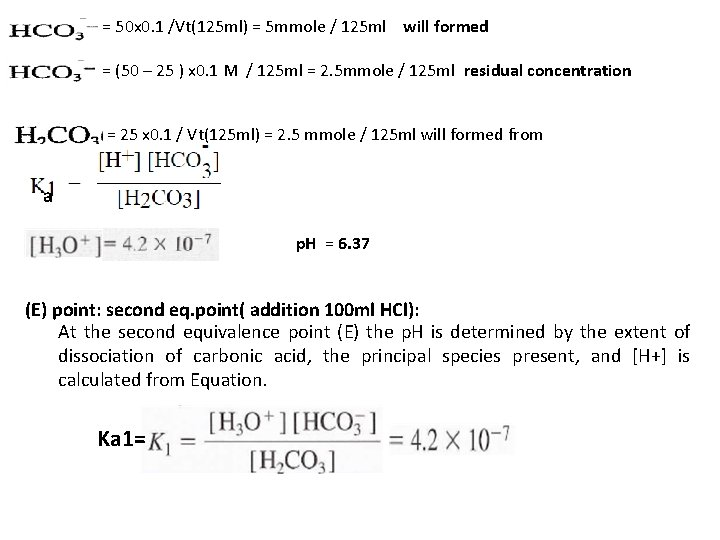= 50 x 0. 1 /Vt(125 ml) = 5 mmole / 125 ml will formed = (50 – 25 ) x 0. 1 M / 125 ml = 2. 5 mmole / 125 ml residual concentration = 25 x 0. 1 / Vt(125 ml) = 2. 5 mmole / 125 ml will formed from a p. H = 6. 37 (E) point: second eq. point( addition 100 ml HCl): At the second equivalence point (E) the p. H is determined by the extent of dissociation of carbonic acid, the principal species present, and [H+] is calculated from Equation. Ka 1=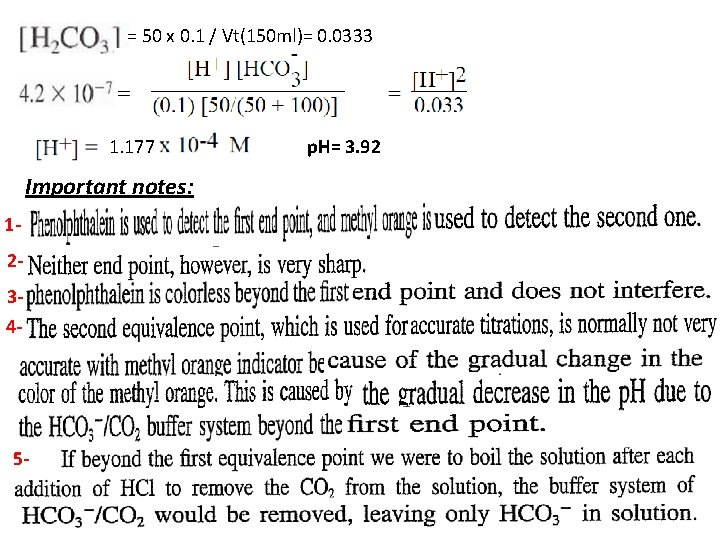= 50 x 0. 1 / Vt(150 ml)= 0. 0333 1. 177 Important notes: 1234 - 5 - p. H= 3. 92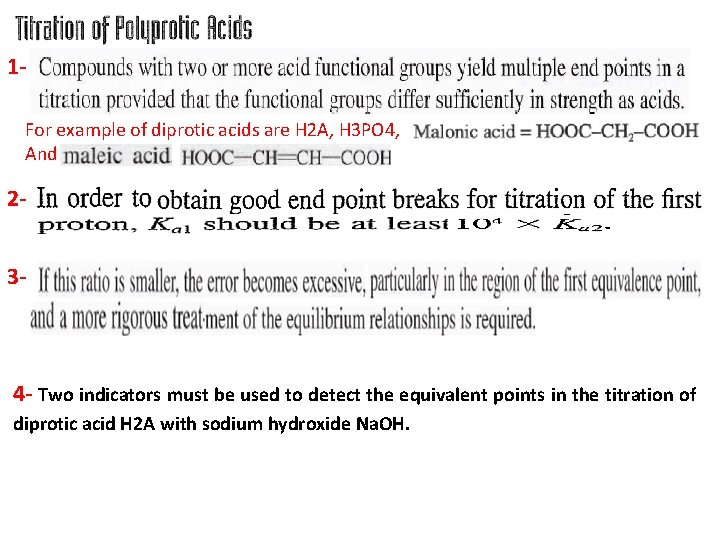1 For example of diprotic acids are H 2 A, H 3 PO 4, And 23 - 4 - Two indicators must be used to detect the equivalent points in the titration of diprotic acid H 2 A with sodium hydroxide Na. OH.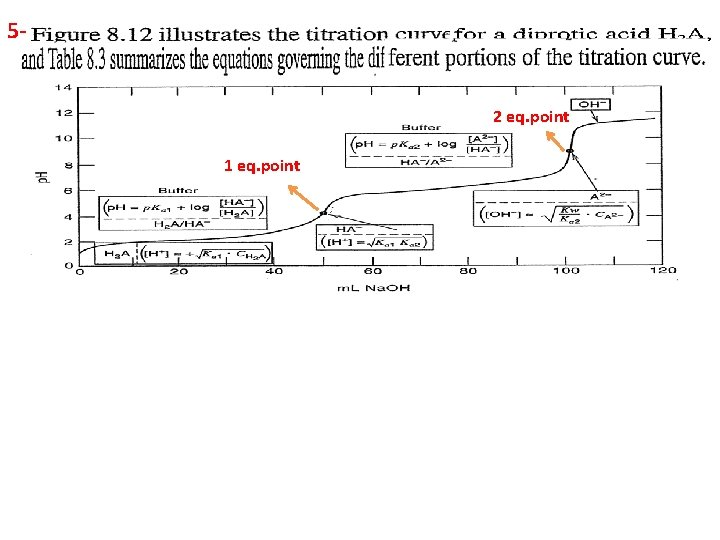5 - 2 eq. point 1 eq. pointExample: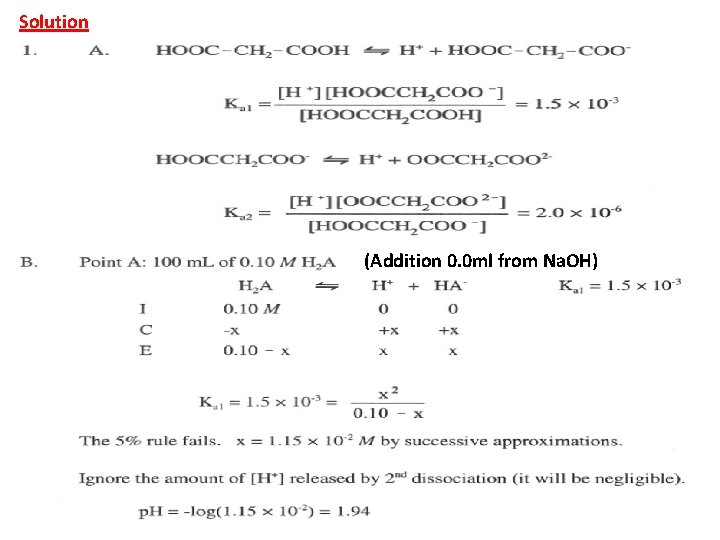Solution (Addition 0. 0 ml from Na. OH)(After addition 50 ml from Na. OH), in this case the solution contains both HA- and H 2 A which is buffer solution: [HA-] = 50 x 0. 1 / Vt(150 ml)= 0. 033 Molar [H 2 A] = (100 – 50) x 0. 1 / Vt(150 ml) = 0. 033 M [HA-] = [ H 2 A] p. H = p. Ka 1 + log HA- / H 2 A p. H = p. Ka 1= 2. 82 (After addition 100 ml from Na. OH) In this case all H 2 A was converted to HA-, which is mono-protic salt and we can calculate the p. H from the above law or.After addition 150 ml from Na. OH), in this case 100 ml from Na. OH will convert all H 2 A to HA-, and the other 50 ml will convert an equal amount from HA- to A-2. Which is buffer solution contains A-2 / HA- and we can calculate the p. H as: [HA-] formed = 100 ml x 0. 1 / Vt( 250 ml) = 0. 04 M [HA-] remaining or residual = (100 – 50) x 0. 1 / Vt(250 ml) = 0. 02 M [A-2] formed = 50 x 0. 1 / Vt(250 ml) = 0. 02 M [HA-] = [A-2] p. H = p. Ka 2 + log [A-2] / [HA-] = 5. 70After addition : 0. 0 ml , 5 ml, 24. 9 ml, 25 ml(first eq. point), 25. 01 ml , 25. 5 ml , 49. 9 ml and 50 ml(second eq. point) from Na. OH.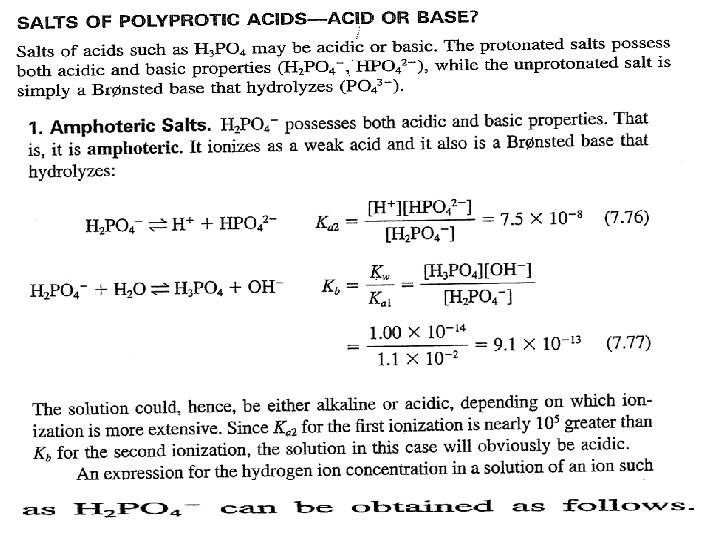So when: 1 - 2 - If and [HA-] / Ka 1 ≤ 13 - If Ka 2× [HA- ] ≤ Kw and [HA-] / Ka 1 >> 1 Kw is not negligible 1 is negligible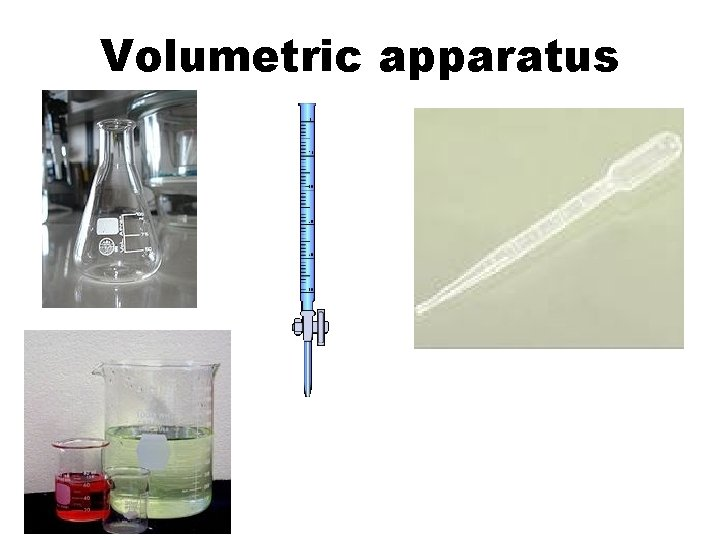Volumetric apparatus